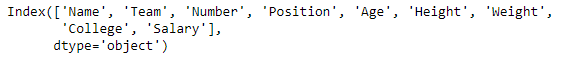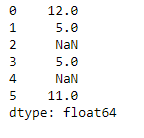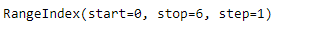# Python | Pandas dataframe.keys()

• Difficulty Level : Basic
• Last Updated : 19 Nov, 2018

Python is a great language for doing data analysis, primarily because of the fantastic ecosystem of data-centric python packages. Pandas is one of those packages and makes importing and analyzing data much easier.

Pandas` dataframe.keys()` function returns the ‘info axis’ for the pandas object. If the pandas object is series then it returns index. If the pandas object is dataframe then it returns columns. If the pandas object is panel then it returns major_axis.

Syntax: DataFrame.keys()

Example #1: Use `keys()` function to find the columns of a dataframe.

 `# importing pandas as pd``import` `pandas as pd`` ` `# Creating the dataframe ``df ``=` `pd.read_csv(``"nba.csv"``)`` ` `# Print the dataframe``df``# find the keys of the dataframe``df.keys()`

Output :Example #2: Use `keys()` function to find the index of the pandas series object.

 `# importing pandas as pd``import` `pandas as pd`` ` `# Creating the series ``sr ``=` `pd.Series([``12``, ``5``, ``None``, ``5``, ``None``, ``11``])`` ` `# Print the series``sr``# to find the index``sr.keys()`

Output :My Personal Notes arrow_drop_up